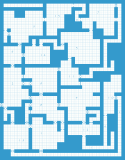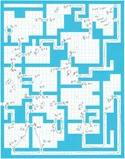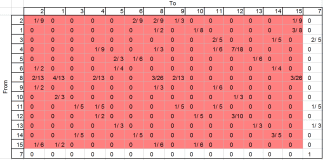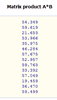Like Most GMs, you probably find your self wondering from time to time just how competent your players are. Are they the well oiled, expertly min-maxed team they claim to be, or are they a bunch of bumbling monkeys? Luckily there’s a simple mathematically accurate method to test their claims based on simple linear algebra:Once you have your map you’ll have to decide where the entrance is, which room is the goal room, and then mark up each room on the map with the probabilities the PCs would randomly move from each room to another. Use your best judgment for this.Â  In this example, I assume that PCs have twice the chance to utilize an obvious exit over a locked, portcullised, or secret exit.Once this quick and easy process is finished, the data needs to be input into a matrix. The matrix should have a row AND a column for each room. The entrance room should be the first row/column and the goal room should be last.Â  Each row should contain the probabilities mapped out in the previous step.Â  For example, if a party in room 12 has probability 1/2 to travel to room 4, 1/5 to travel to room 10, and 3/10 to travel back to room 12 itselfÂ  (in this case because two of the possible exits from the room lead to obstructed paths that the PCs may not overcome) then the 12th row would have the following values: 0 0 0 1/2 0 0 0 0 1/5 0 3/10 0 0 0 0.Â  Regardless of probabilities mapped out in the previous step, the goal room should have a row of zeros with a single 1 in it’s own column. This represents the fact that once the PCs have achieved this room that they’re done.

Of particular interest is the highlighted sub-matrix which excludes the row and column from the goal room.Â  This sub-matrix is denoted Q, and is the portion of our matrix on which we’ll actually be performing matrix algebra.

Speaking of which, we’re now going to want to calculate (I-Q)^-1 * R where I is a standard identity matrix of the appropriate dimensions, ^-1 indicates and inverse matrix function, and R is a standing vector of 1s of appropriate dimension.Â  If you need help with that, head over to http://www.bluebit.gr/matrix-calculator/ which is an excellent web-based matrix calculator.You’ll first want to select their Matrix Addition/Subtraction tool and insert your identity matrix into in the input box for matrix A (an identity matrix is a matrix of all 0s, except for the elements on the diagonal, which are 1s. In this case you want it to have the same number of rows and columns as your Q matrix) and your Q matrix into the input box for matrix B.

After you click the “Subtract A-B” button, you’ll get a results page where you can verify your two matrices and see the result of your operation.Â  Select and copy the output matrix, which is (I-Q) then go back to the matrix calculator.Once there, you’ll want to select the “Matrix Inverse” checkbox and paste your (I-Q) matrix into the input box and click “calculate”.Â  That will take you to a results page where you can select and copy your inverted matrix (I-Q)^-1 then return to the matrix calculator for a final step.Finally, select the “Matrix Multiplication” tool and paste your (I-Q)^-1 matrix into the input box for matrix A.Â  For Matrix B, enter a single column of 1s with the same number of rows as your (I-Q)^-1 matrix. Then click the “Multiply A*B” button.This willÂ  result in an output matrix that’s a standing vector with a single column and the same number of rows as your B matrix, but you’re only interested in the very first element in this matrix (which is why we re-arranged the rows and columns of our original matrix to put your entrance room in the first row/column.Â  For our example, this number is 54.349.

So what is that number?Â  That’s the average number of (non-unique) rooms a party will travel through before finding the goal room if they travel completely randomly through the dungeon.Â  The larger and more complex the dungeon, and the more difficult optimal paths are to take, the larger this number will grow. Of course, this number assumes totally random movement so it assumes a certain amount of stupidity on the part of your players.Â  In room 14 of our dungeon for example, we see the party has a 60% chance to exit via the closet door in the north wall and then return (the other doors are either locked or the door in which they came) but the random movement doesn’t assume that once they’ve tried that door they won’t try it a few more times just to be sure. In fact, with a 60% probability attached to it, they probably do try it a few times. Of course, we’ve all seen our players do the exact same thing, so it’s probably not so bad a model after all.

With a quick glance at your map, you can also see the minimum number of rooms the party will have to travel through to reach the goal room.Â  In our example, this is 4.

Using these two numbers as guidelines, you can easily gauge your player’s performance through your dungeon.Â  If they can’t beat the average completely random number of rooms, they’re actually doing WORSE than if they simply rolled dice to decide what to do.Â  If they do better than the optimal number, they’re bloody cheaters, but it’s the numbers in the middle that are the most interesting.